Compose/Decompose
Name that Fraction!
Equivalent Fractions
Comparing
Mystery
100

Draw a circle model for the fraction  3/4.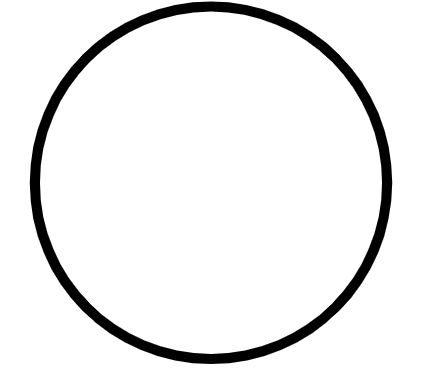3/4  is four equal parts with three parts shaded.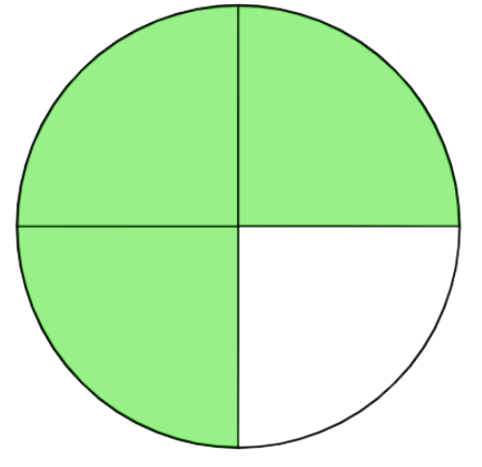100

What fraction is represented by the point on the

number line?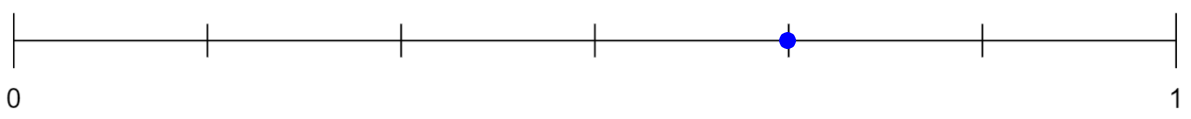4/6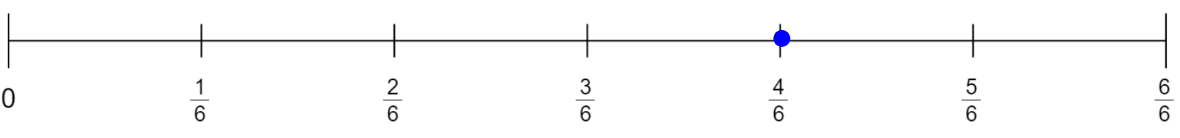100

Which model is equivalent to 2/3?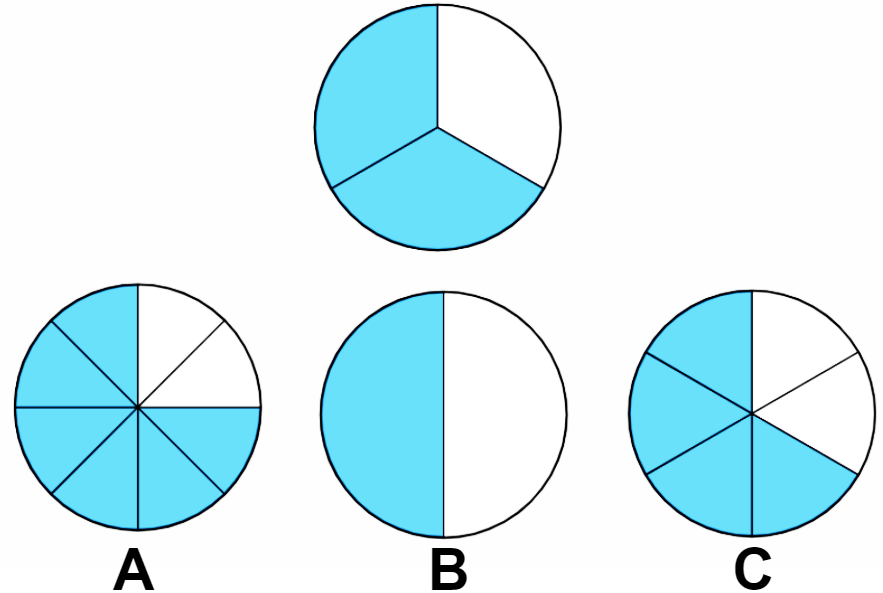2/3 = 4/6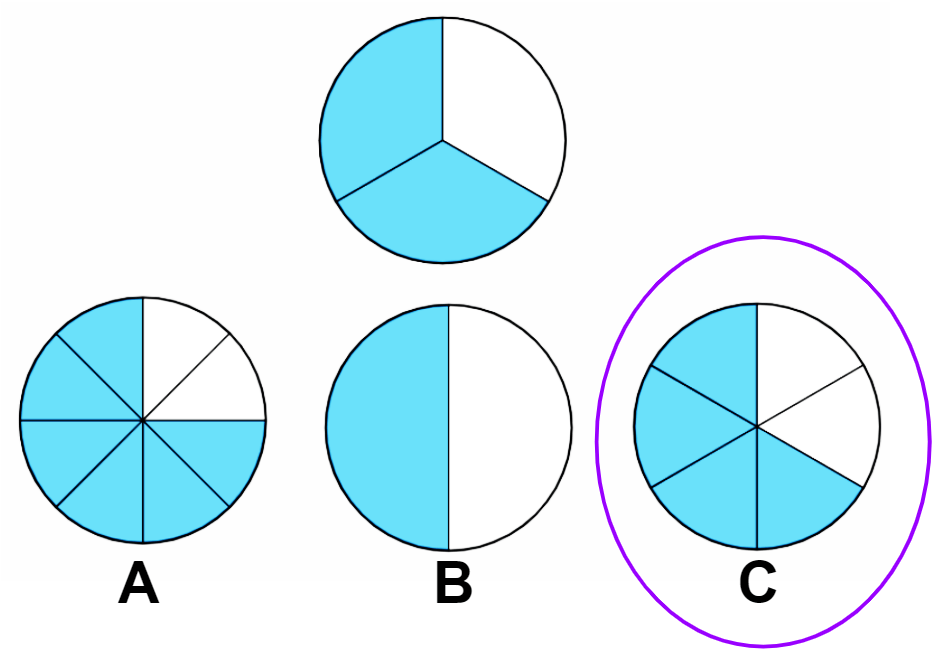100

Which comparison is true?

1/8 > 1/4              2/3 > 2/6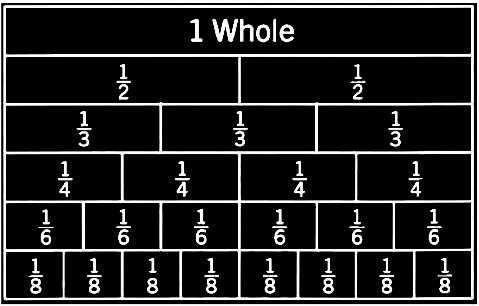2/3 > 2/6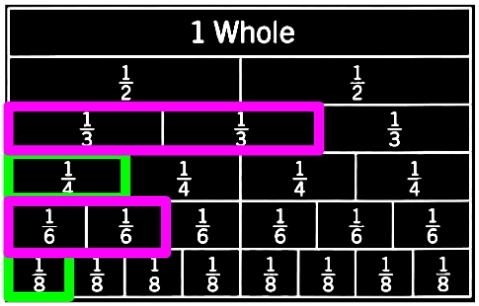100

Name the fraction shown below.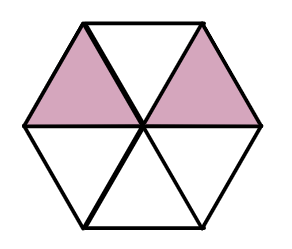2/6200

Use a rectangle to compose a model for the sum of unit fractions below.
1/6 + 1/6 + 1/6 + 1/6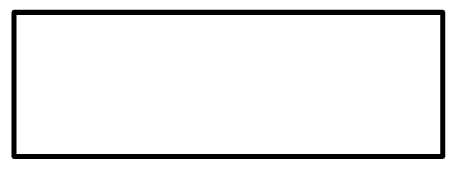1/6 + 1/6 + 1/6 + 1/6 = 4/6200

Jose wants to label  1/3   on the number line.  At which point should Jose label   1/3 ?Point B =  1/3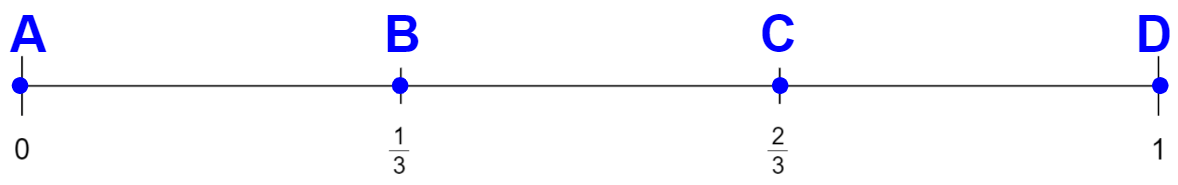200

Which two models show equivalent fractions?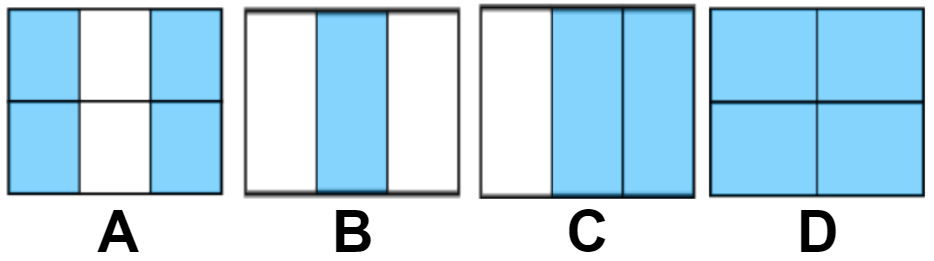4/6 = 2/3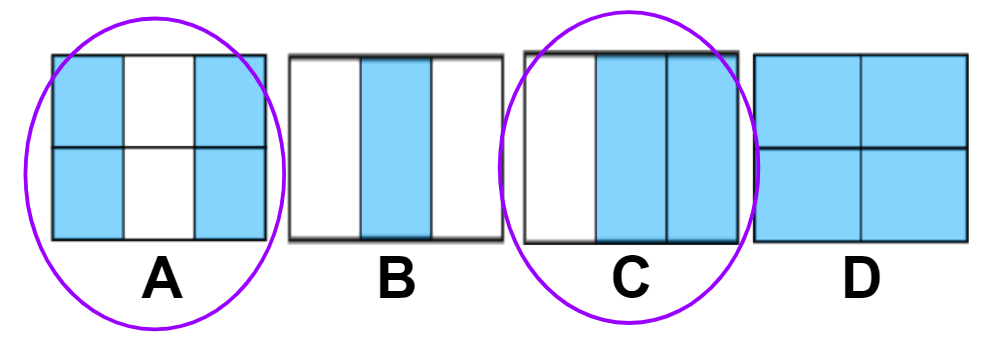200

Complete the statement comparing the model.____ > ____ because _______ are larger equal parts than ______.

Complete the statement comparing the model.3/3  >  3/8 because thirds are larger equal parts than eighths.

200

The picture below represents the juice boxes three sisters brought to school.  Each sister brought the same number of juice boxes.  What fraction of the juice boxes did each sister bring?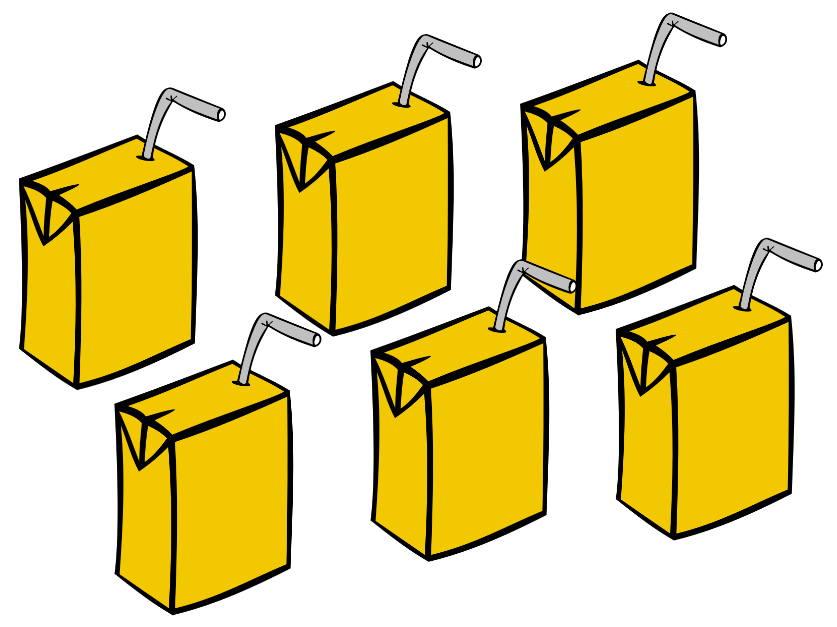6 juice boxes shared among 3 people = 2 juice boxes each.  They each received  2/6  of the juice boxes.

1/6 + 1/6 = 2/6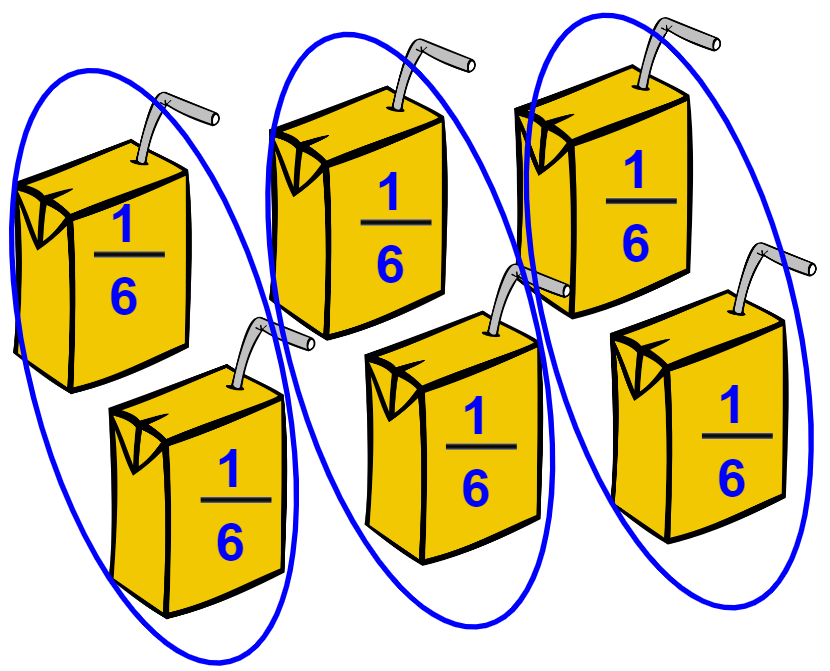300

Jesse's little brother ate  2/8  of his sandwich.  Write  2/8  as a sum of unit fractions.

2/8 = 1/8 + 1/8

300

What fraction is represented by the point on the

number line?7/8300

According to the number line, which fraction is equivalent to

6/8

?3/4  =  6/8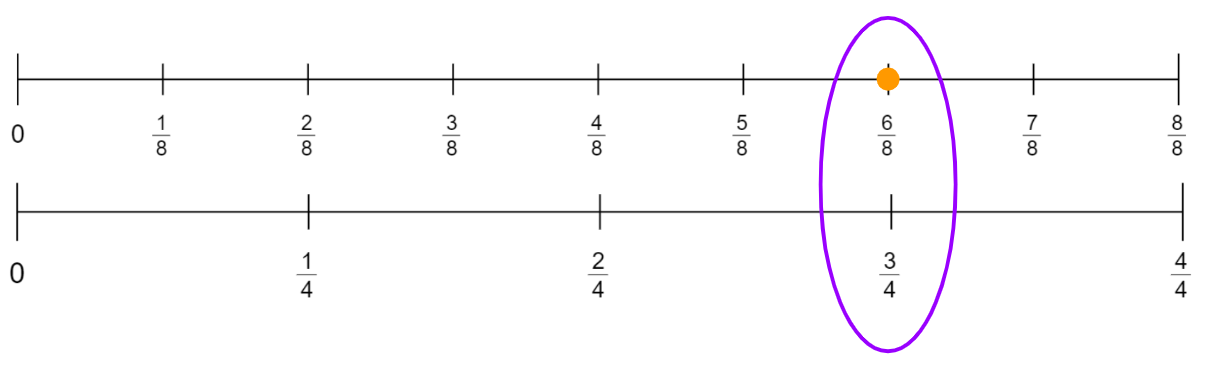300

Compare the following fractions.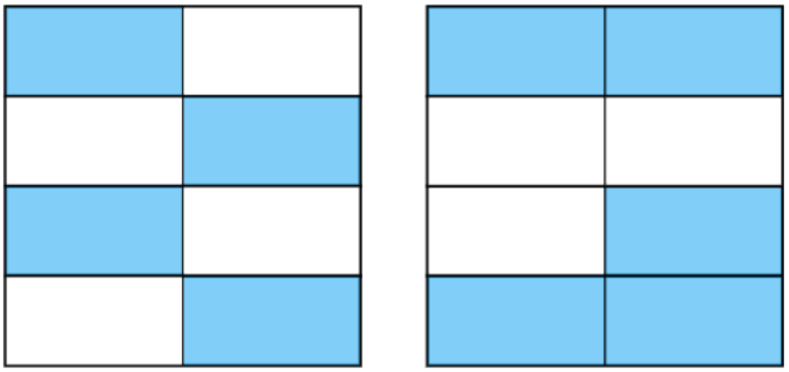4/8 < 5/8   or   5/8 > 4/8300

Compare the fractions shown.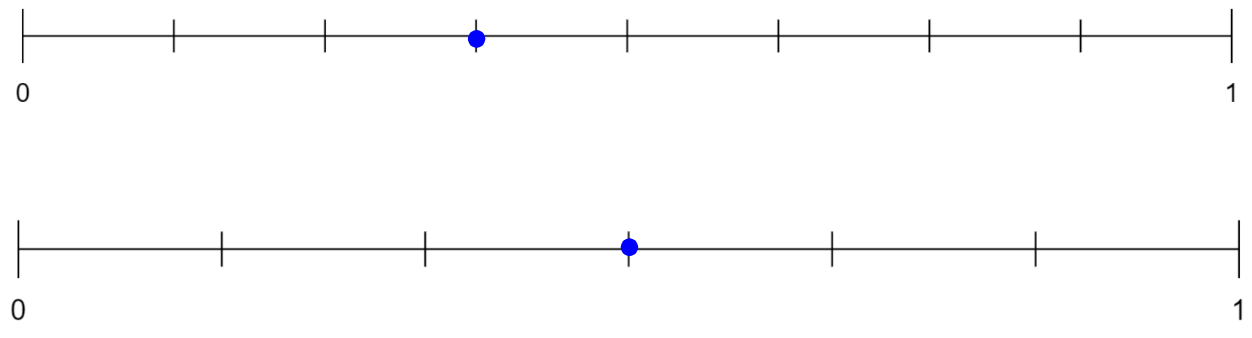3/8 < 3/6   or   3/6 > 3/8400

Victoria says the two models below are equivalent fractions.  Is Victoria correct?  Why or why not?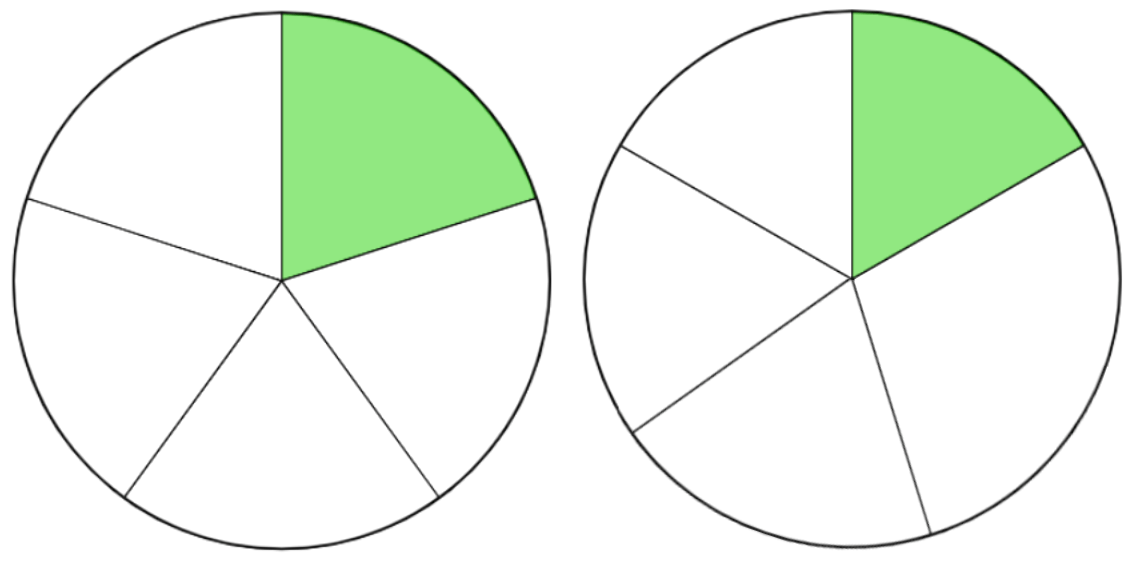Victoria is incorrect.  The first fraction is  1/5 .  There are 5 equal parts with 1 part shaded.  The second fraction can't be  1/5  because the parts are not equal in size.400

What fraction is represented by the model below?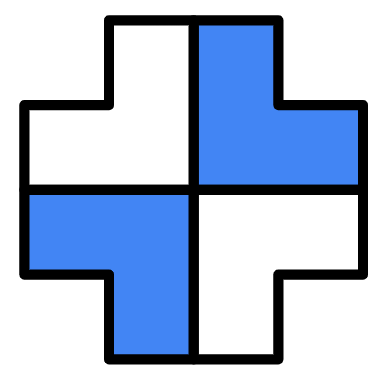2/4     or      1/2400400

Compare the fractions shown.1/6 < 3/6   or   3/6 > 1/6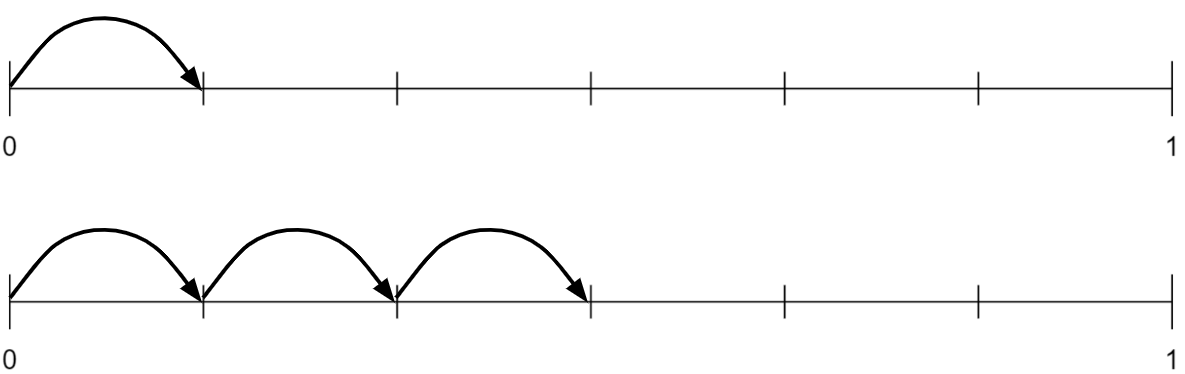400

Tam and Lee shared the Chinese dumplings pictured below. What fraction of dumplings did they each receive?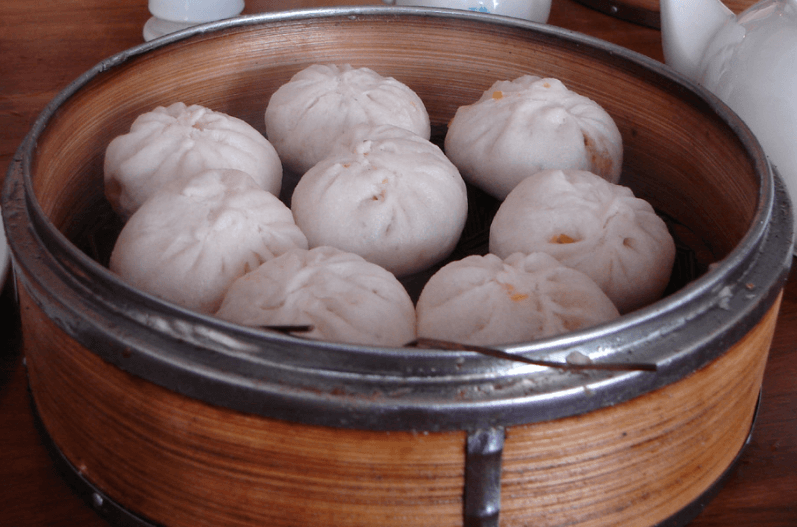8 dumplings shared among 2 people = 4 dumplings each.  They each received  4/8  of the dumplings.

1/8 + 1/8 + 1/8 + 1/8 = 4/8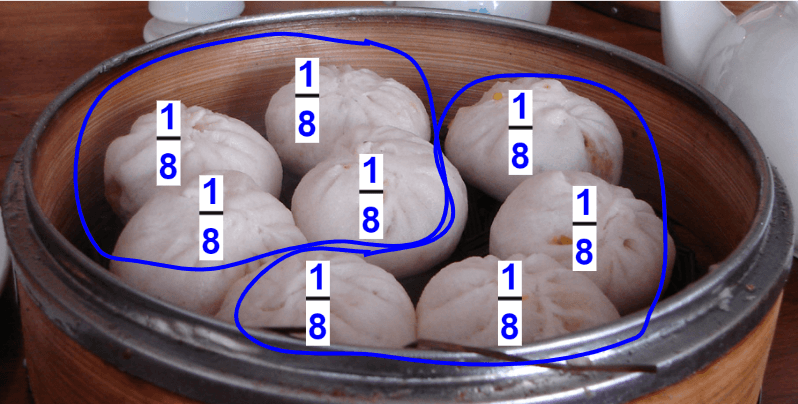500

There are 6 Lego figures displayed. Write an addition expression that represents the number of Lego figures with red shirts.1/6 + 1/6 + 1/6 +1/6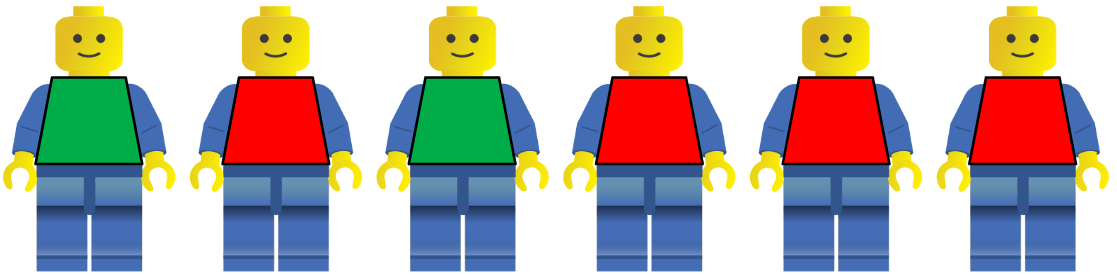500

What fraction of the sugar cookies have blue icing?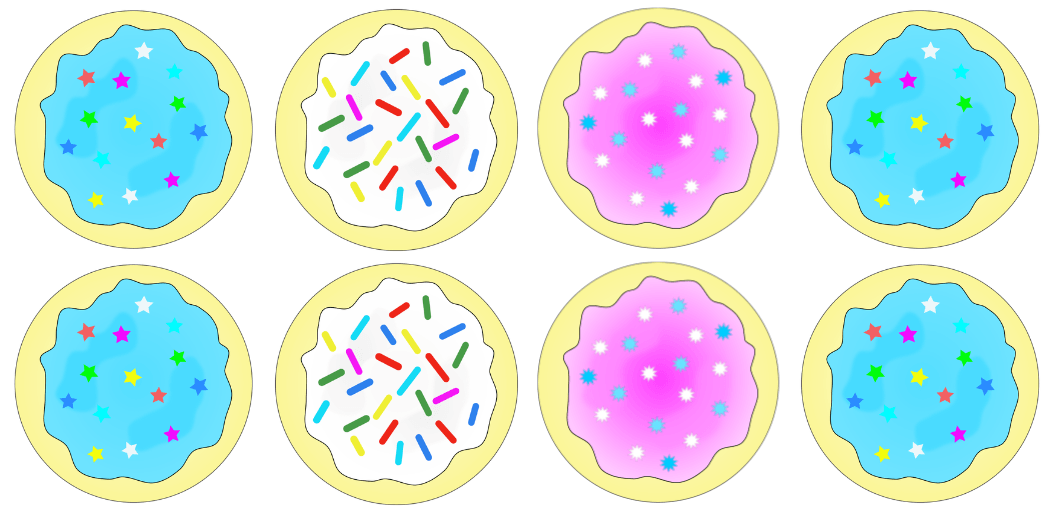4/8    or    1/2500

What two fractions can represent the fraction of triangles shaded?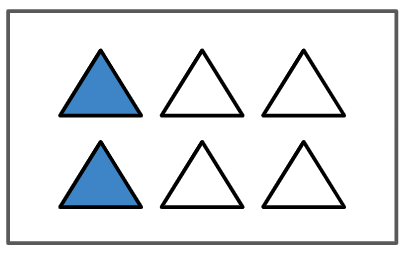1/3 and  2/6500

Complete the statement comparing the model.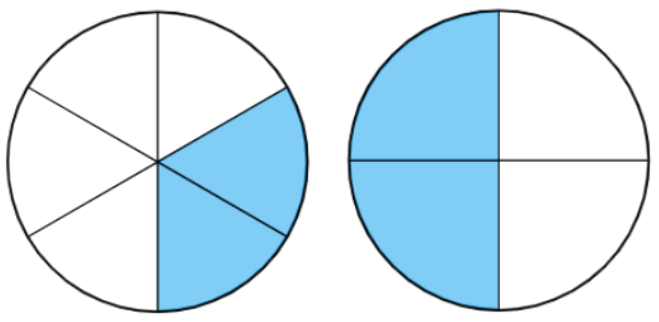____ > ____ because _______ are larger equal parts than ______.2/4 > 2/6  because fourths are larger equal parts than sixths.

500

Name any fraction equivalent to  1/2

1/2 = 2/4 = 3/6 = 4/8

Teachers can choose to accept fractions with other denominators as long as they represent

1/2

Click to zoom